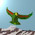## Saturday, March 26, 2011

### Theory Update 75

Recall that the Bilson-Thompson braids are naturally extended by the mirror neutrino states. One generation is shown here.The mirror composition of a left handed up quark diagram with a right handed one results in one of the $(123)$ braided permutations. When charge is represented by the cubed roots, two full positive twists become a negative twist. Thus the diagram on the right should be associated to a charge $- 2/3$ boson. Similarly, the down quark mirror composition yields a charge $+ 1/3$ diagram.

Note that whereas the net neutral charge of a mirror $Z$ boson diagram came from the over under crossings of a fermion braid, one can obtain a $2/3$ charge in $B_3$ by composing a mirror pair of over over diagrams. One requires three copies of such a diagram to obtain an integral charge braided $W^{\pm}$ diagram, with $12$ crossings. In order to understand, say, pion decay, we may need to look at both the $B_3$ and $B_6$ representations of bosons. Such a decay mixes the charges of the two quarks, and yields a charged lepton and neutrino. This is easily drawn using a $B_6$ diagram, but there is some redundancy in using so many strands just to interchange two $1/3$ charges.

1.Oh, look! The loopies have discovered a new Principle: Relative Locality! Yeah, right, like they are the first to think of that. WTF do they think all the non stringers and loopies have been thinking about for 20 years.

2.The loopies now predict GRB time delays based on nonlocality for distant observers. Here are a couple of old posts where we mention this idea:

1. On GRB 090510
2. Another blurb.

3.4.The principle of relative locality
http://arxiv.org/abs/1101.0931

No spacetime, but a phase space! This is a ZEO?

5.Ulla, no, they don't seem to understand the ZEO, although they are getting closer. At present they are 'fixing scales' and looking at infrared QG effects by letting $\hbar$ go to zero. So they might eventually appreciate Louise's law, but they will probably make up a big confusing story about how it was all their idea.

6.Yes, I could not understand their Planck mass equation. The c has disappeared but I saw no explanation.

7.As I see it, without carefully reading the paper, but assuming they are making vague sense, they are essentially fixing $c$ (separately) in the local physics at the two locations of the GRB thought experiment. I think this is more or less OK, for the situation they are discussing, but of course the missing explanation needs to be provided eventually.

Morally, they should perhaps be taking $c \rightarrow \infty$ in their scheme, since $\hbar \rightarrow 0$. But Riofrio's law can still be enforced by assuming that $t \rightarrow 0$ instead, for fixed $M$ and $c$, which is correct when $\hbar \rightarrow 0$.

8.Here is Ulla's long post on relative locality.

Note: Only a member of this blog may post a comment.